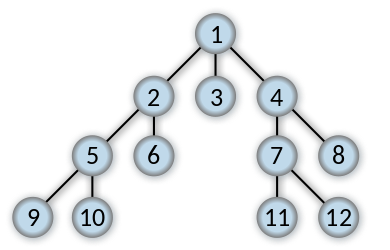If you have or had troubles understanding breath or depth first search, you’ve come to the right place. Here it is – recursive graph search made easy.

### Depth-First Search

Depth-first search (DFS) is a method for exploring a graph. In a DFS, you follow the child of traversing node, meaning if the current node has a child, with each step you’ll go deeper into the graph in terms of distance from the root. If a node hasn’t any unvisited children, then go up one level to parent.Breadth-first search (BFS) is a method for exploring a graph. In a BFS, you first explore all the nodes one step away from the root, then all the nodes two steps away, etc.### The Problem

Consider a matrix with rows and columns, where each cell contains either a ‘0’ or a ‘1’ and any cell containing a 1 is called a filled cell. Two cells are said to be connected if they are adjacent to each other horizontally, vertically, or diagonally. If one or more filled cells are also connected, they form a region. find the length of the largest region.

``````Input : M[] = { 0 0 1 1 0
1 0 1 1 0
0 1 0 0 0
0 0 0 0 1 }
Output : 6
Ex: in the following example, there are 2 regions one with length 1 and the other as 6.
The largest region : 6``````

### The Solution

How to tackle the problem? We cannot simply iterate through the two-dimensional array, because whenever we find a positive value, we should be defining constraints dynamically and while that is definitely possible, it would not be a short nor an elegant solution. Fortunately, aforementioned graph traversal techniques come to the rescue.

##### DPS Solution

First, we define the search area engine by using two simple loops (for iterative solution instead of recursive we could use a stack):

``````public static int getBiggestRegion(int[,] matrix)
{
int maxRegion = 0;

for (int row = 0; row < matrix.GetLength(0); row++)
{
for (int column = 0; column < matrix.GetLength(1); column++)
{
if (matrix[row,column] == 1)
{
int size = getRegionSize(matrix, row, column);
maxRegion = Math.Max(size, maxRegion);
}
}
}

return maxRegion;
}``````

This function iterates through the entire matrix and for every point [row, column] executes the getRegionSize function (we’ll get to that in a second), simple as that. The Math.Max is added to check if the current result is bigger than the biggest result found so far.

``````private static int getRegionSize(int[,] matrix, int row, int column)
{
if (row < 0 || column < 0 || row >= matrix.GetLength(0) || column >= matrix.GetLength(1))
{
return 0;
}
if (matrix[row, column] == 0)
{
return 0;
}

matrix[row, column] = 0;
int size = 1;
for (int r = row - 1; r <= row + 1; r++)
{
for (int c = column - 1; c <= column + 1; c++)
{
if (r != row || c != column)
size += getRegionSize(matrix, r, c);
}
}
return size;
}``````

Ok, let’s have a look at getRegionSize. Because we’re going to use it recursively, the exit conditions are defined at the beginning i.e. matrix size boundaries + the condition for the positive value of the point.

The matrix[row, column] = 0; is important. It is our marking mechanism for visited coordinate. This avoids going through the same elements. It has a side effect, though – it’s destructive for the iterated array, so in order to avoid it, we would need to clone the array in the first place. On the contrary, cloning requires additional memory.

Then for every point adjacent to the current point getRegionSize function is executed recursively. So if there are no more ‘1’ next to the last point, the function will return 1 and then add +1 for every execution that was put on a stack, thus calculating the largest positive region in the matrix.

##### BFS Solution

Notice that in the DFS solution we followed a point containing ‘1’ immediately after it was found. In the Bread-First search we want to have a look at the nearest neighbor of each point first. Also, we’re going to use nondestructive method with queue and an additional hashset of visited coordinates.

Nothing changes in the getBiggestRegion method but, we need to add two properties:

``````private static Queue<Tuple<int, int>> coordinates = new Queue<Tuple<int, int>>();
private static HashSet<Tuple<int, int>> visited = new HashSet<Tuple<int, int>>();``````
``````private static int getRegionSizeBFS(int[,] matrix, int row, int column)
{
if (row < 0 || column < 0 || row >= matrix.GetLength(0) || column >= matrix.GetLength(1))
{
return 0;
}
if (matrix[row, column] == 0 || visited.Contains(new Tuple<int, int>(row, column)))
{
return 0;
}

int size = 1;
for (int r = row - 1; r <= row + 1; r++)
{
for (int c = column - 1; c <= column + 1; c++)
{
if ((r != row || c != column) && !visited.Contains(new Tuple<int, int>(r, c)))
{
coordinates.Enqueue(new Tuple<int, int>(r, c));
}
}
}

while (coordinates.Any())
{
var point = coordinates.Dequeue();
size += getRegionSizeBFS(matrix, point.Item1, point.Item2);
}
return size;
}``````

We are using the queue to store the coordinates of the nearest (with max distance of 1 square for each point). Then if there are no more items in the queue, we get the first element from it and execute the function one more time.

Here are the full listings:

DFS

BFS